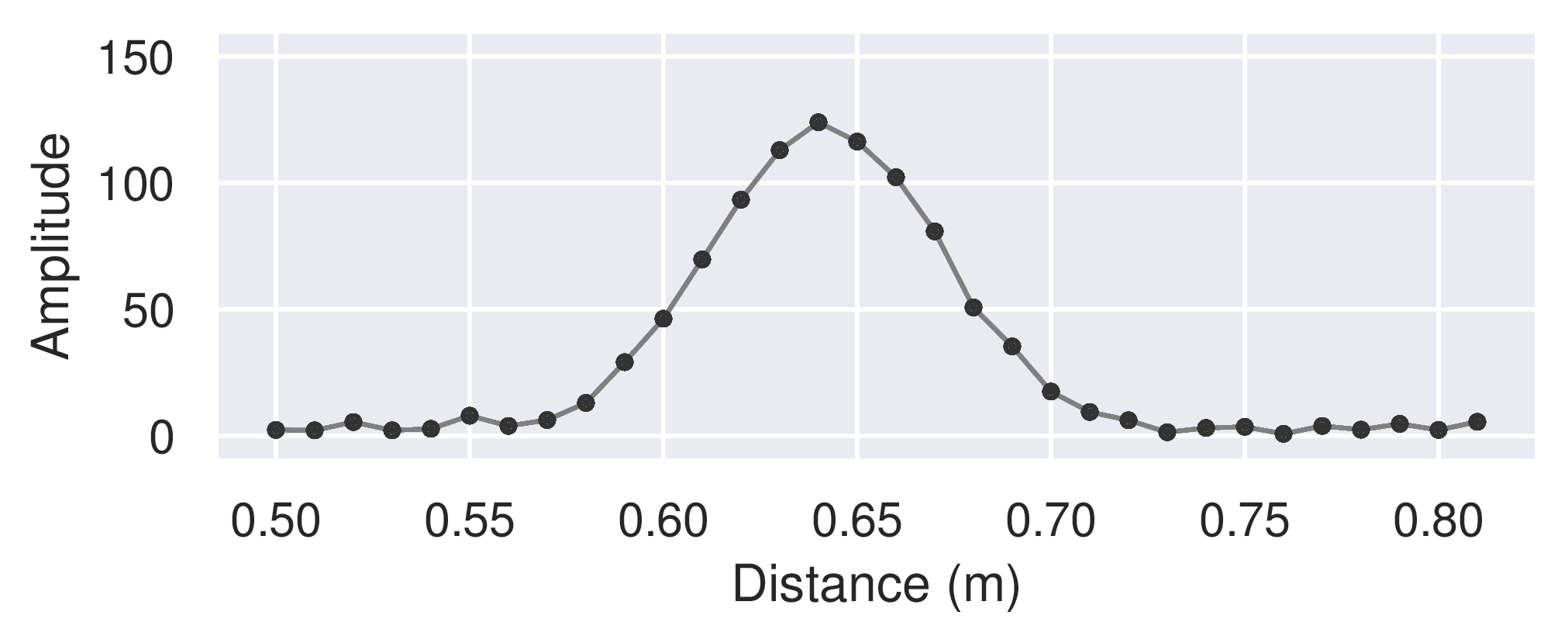# Measurement range#

The sparse IQ service can be configured to measure practically any range of distances in a so-called sweep. In other words, a sweep makes up the points in space where reflected pulses are measured.Figure 5 Amplitude of a mocked sweep of an environment with a single object.#

Figure 5 above shows an example of a sweep with a range spanning from a start point at ~ 0.50 m to an end point at ~ 0.81 m. Here, a total of 32 points were measured with a distance between them of ~ 10 mm, also configurable. The shortest configurable distance between points is ~ 2.5 mm.

The more points that are measured, the more memory is used and the longer it takes to measure the sweep. This may in turn lead to higher overall duty cycle and power consumption. Thus, it is often important to try to minimize the number of points measured, typically achieved by maximizing the distance between the points.

## Configuration#

For preciseness, the range is configured in a discrete scale with three integer parameters – the start point $$d_1$$, the number of points $$N_d$$, and the step length $$\Delta d$$. The step length corresponds to the distance between the points (mentioned above). The distance between the points in the discrete scale is ~ 2.5 mm, which is also why the shortest configurable distance between points is just that.

Figure 6 above demonstrates how a range can be set up with these parameters. The start point $$d_1=20$$, the number of points $$N_d=4$$, and the step length $$\Delta d = 3$$ This gives the discrete points $$\{20, 23, 26, 29\}$$ which correspond to $$\{50.0 mm, 57.5 mm, 65.0 mm, 72.5 mm\}$$.

Note that the possible values for step length $$\Delta d$$ are limited.

## Limitations#

The only limitation on the number of points $$N_d$$ itself is related to the available buffer size of 4095 complex numbers. The buffer usage is the number of points $$N_d$$ times the number of sweeps per frame $$N_s$$ (see Frames, sweeps and subsweeps). In short, $$N_d \cdot N_s \leq 4095$$.

The step length must be a divisor or multiple of 24. The shortest step length, 1, gives a distance between points of ~ 2.5 mm. See Table 2 for an overview.

Table 2 Overview of selectable step lengths.#

Step length

Step length cont’d

Setting $$\Delta d$$

Distance

Setting $$\Delta d$$

Distance

1

2.5 mm

24

60 mm

2

5.0 mm

48

120 mm

3

7.5 mm

72

180 mm

4

10.0 mm

96

240 mm

6

15.0 mm

120

300 mm

8

20.0 mm

144

360 mm

12

30.0 mm

The maximum measurable distance (MMD), i.e., the farthest configurable “end point”, is limited by the pulse repetition frequency (PRF). The lower PRF, the longer MMD.

The PRF also gives the maximum unambiguous range (MUR) – the maximum range at which target can be located at while still guaranteeing that the reflected pulse corresponds to the most recent transmitted pulse. Again, the lower PRF, the longer MUR.

See Frames, sweeps and subsweeps on the PRF for more details.

## Caveats#

A number of factors affect the actual real world distance of a given range point:

• The refractive index and thickness of materials the radar signal pass through.

• Systematic errors due to process, supply voltage, and temperature variations.

• Reference clock frequency.

Some static offsets can be compensated for by doing a loopback measurement of the “zero point”.